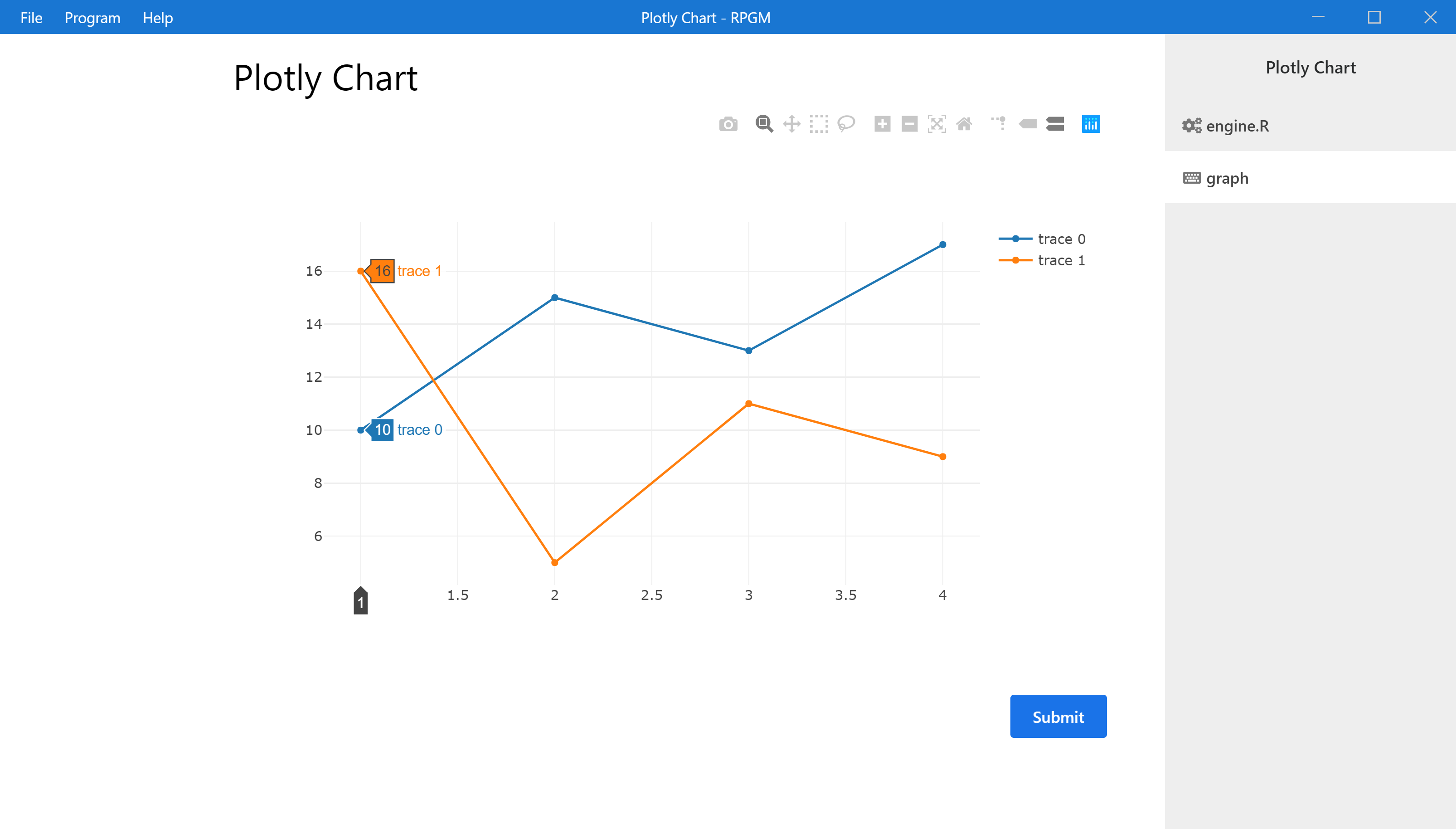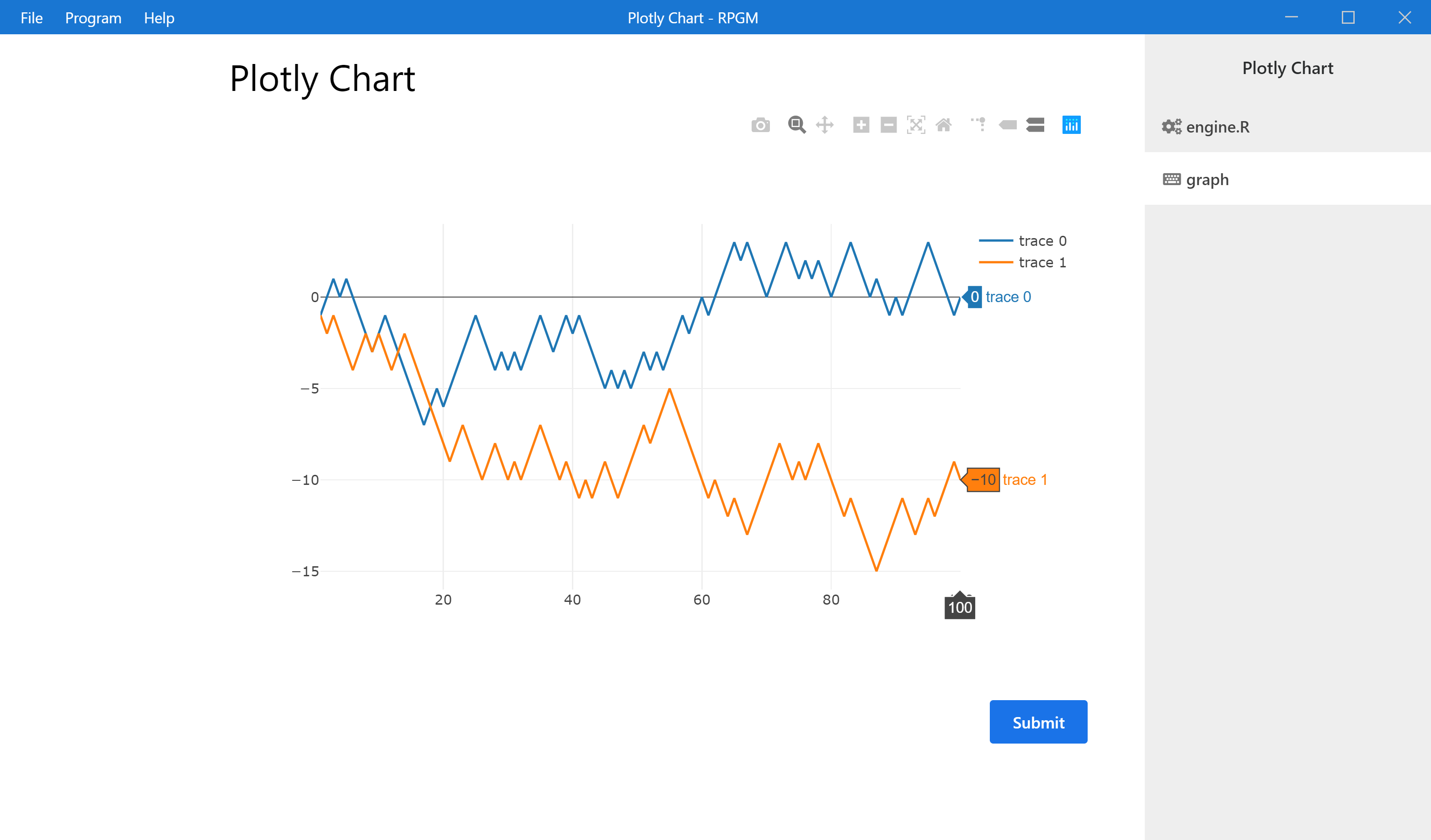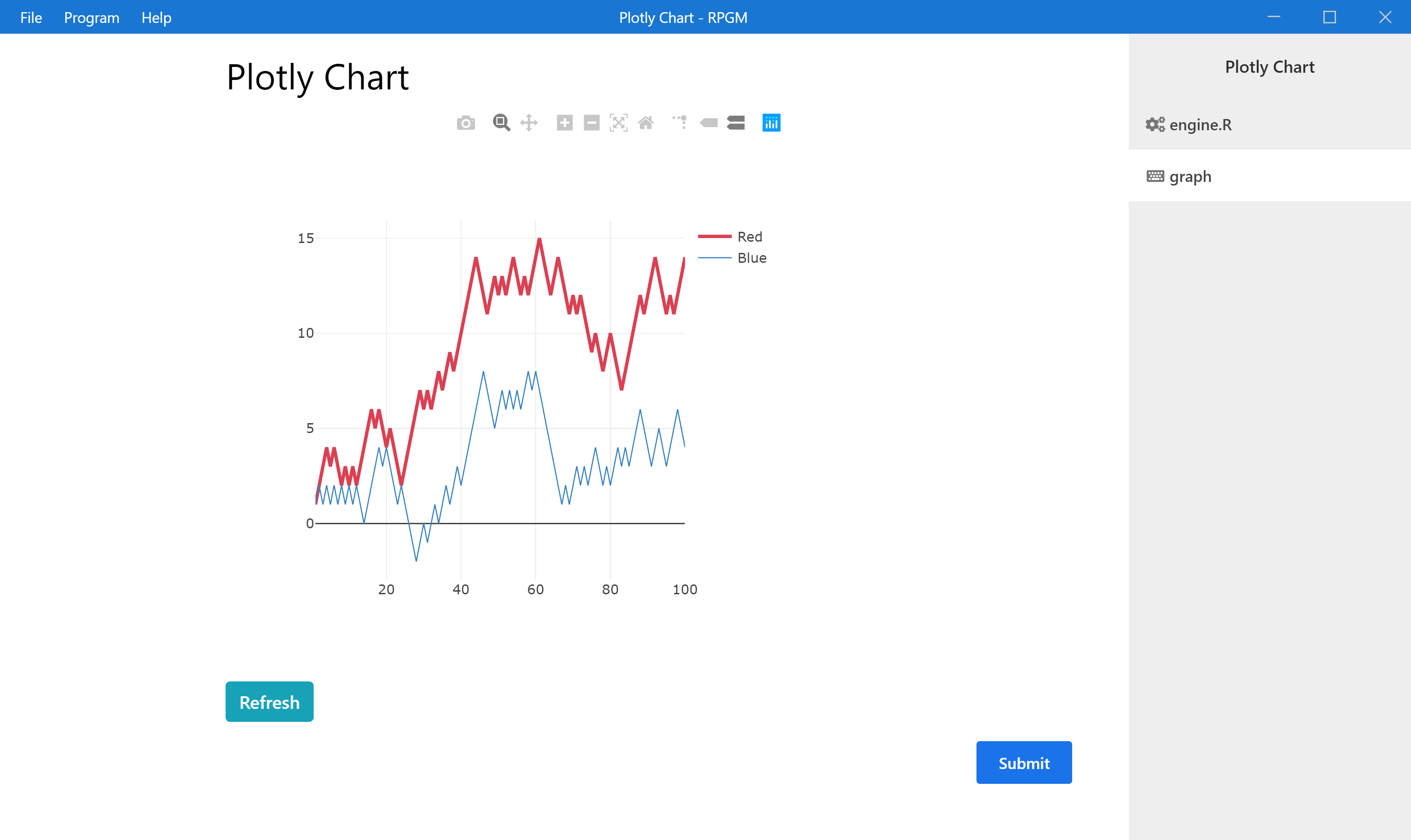# Plotly

The aim of this tutorial is to understand how to build plotly graphics in RPGM using the widgets graph. The widget graph is designed to get an R variable (a list) that represents the plotly chart.

How does it works ? RPGM directly includes plotly natively from the javascript. It takes the same arguments as the javascript version but as an R list. Don't worry, you will not need to learn anything about javascript, it is actually very simple, let's start with an example!

Create a new project with the File menu, then New project..., select RPGM project and name our new project "ChartsWithPlotly".

## A first graph

We begin with a simple line chart. We take the example given by the plotly help and we then adapt it in order to provide a custom chart, with a random walk.

Create a GUI file named graph.pgui, and add it to your sequencer. In the GUI file, add the following widgets:

• A Label (that you can customize),
• A Graph.

In the widget Graph, fill the field Graph variable with `mygraph`. It is the name of a Graph variable that we will build. Create an R script named engine.R and add it to the sequencer before the GUI step.

We will display a simple line chart with plotly. In the Javascript help at https://plot.ly/javascript/line-charts/, we have the following code in javascript:

``````var trace1 = {
x: [1, 2, 3, 4],
y: [10, 15, 13, 17],
type: 'scatter'
};

var trace2 = {
x: [1, 2, 3, 4],
y: [16, 5, 11, 9],
type: 'scatter'
};

var data = [trace1, trace2];

Plotly.newPlot('myDiv', data);
``````

In RPGM, we need to recreate the variable data in the same way, using R lists (and, if possible, we can also use R vectors). In particular, `trace1` will be a list that contains:

• an `x` component, which can be either a list or a vector,
• a `y` component, which can be either a list or a vector,
• a `type` component, which is a simple character's string that gives the style of the line.

We do the same with `trace2` and we create a list with `trace1` and `trace2` which is our `data` variable. Finally, we create the `mygraph` variable as a list with data. To sum up, to display this example, our script in engine.R is:

``````trace1 = list(                      # var trace1 = {
x = c(1, 2, 3, 4),              #   x: [1, 2, 3, 4],
y = c(10, 15, 13, 17),          #   y: [10, 15, 13, 17],
type = 'scatter'                #   type: 'scatter'
)                                   # };

trace2 = list(                      # var trace2 = {
x = c(1, 2, 3, 4),              #   x: [1, 2, 3, 4],
y = c(16, 5, 11, 9),            #   y: [16, 5, 11, 9],
type = 'scatter'                #   type: 'scatter'
)                                   # };

data = list(trace1, trace2)         # var data = [trace1, trace2];

mygraph <- list(data = data)        # Plotly.newPlot('myDiv', data);
``````

Note that, the graph variable is a list that contains a member `data` witch is the name of the variable in the plotly example. See clearly the analogy between the javascript version (in comment above on the right) and the RPGM version (the R code on the left). It always works in the same way! Save and test the program with F5.Now, we customize our graph. We will display two paths of a random walk.

The following function simulates such a random sequence :

``````RW <- function(n)
return(cumsum(1-2*rbinom(n, 1, 0.5)))
``````

Then, we adapt the code of engine.R in order to provide two paths of the random walk with `n = 100` simulations.

``````RW <- function(n)
return(cumsum(1-2*rbinom(n, 1, 0.5)))

n <- 100
trace1 = list(
x = 1:n,
y = RW(n),
type = 'scatter'
)

trace2 = list(
x = 1:n,
y = RW(n),
type = 'scatter'
)

data = list(trace1, trace2)
mygraph <- list(data = data)
``````

Push the F5 key to execute the program.## Update dynamically the graph

Since the paths are random, we can generate them several times. We now add a button in order to resimulate the paths and to refresh the chart. In the graph.pgui file:

• in the widget Graph, add an ID. For example, fill the ID filed with `graphRW`. This is important, since we will update the chart dynamically using the ID.
• add a Button widget. Fill the field Value of the button with `Refresh` and fill the filed R when pressed with `updategraph(100)`. `updategraph` is a function which regenerates the graph and that we define in engine.R (see the code below).

The whole R file is:

``````RW <- function(n)
return(cumsum(1-2*rbinom(n, 1, 0.5)))

n <- 100

generategraph <- function(n)
{
trace1 = list(
x = 1:n,
y = RW(n),
type = 'scatter'
)

trace2 = list(
x = 1:n,
y = RW(n),
type = 'scatter'
)

data = list(trace1, trace2)

return(list(data = data))
}

mygraph <- generategraph(n)

updategraph <- function(n)
{
mygraph <<- generategraph(n)
gui.update("this", "graphRW")
}
``````

Note

The `updategraph` function first modifies the variable `mygraph`, which is called in the Graph variable field of the widget `graphRW`. We modify it globally (outside of the function) with `<<-` and then ask to update the graph with `gui.update(step, id)` which refreshes the field Graph variable / Value of the corresponding widget.

Save and push F5 to execute your program. You can now refresh the graph with two new random paths each time you push your Refresh button.

## Styling the graph

We would like to customize the style of our graph, using more options easily. Look at the Styling Line Plot section for the javascript code, which is

``````trace1 = {
type: 'scatter',
x: [1, 2, 3, 4],
y: [10, 15, 13, 17],
mode: 'lines',
name: 'Red',
line: {
color: 'rgb(219, 64, 82)',
width: 3
}
};

trace2 = {
type: 'scatter',
x: [1, 2, 3, 4],
y: [12, 9, 15, 12],
mode: 'lines',
name: 'Blue',
line: {
color: 'rgb(55, 128, 191)',
width: 1
}
};

var layout = {
width: 500,
height: 500
};

var data = [trace1, trace2];

Plotly.newPlot('myDiv', data, layout);
``````
As we can see :

• There are more options in `trace1` and `trace2`, we just need to add them in the R code
• The particular option `line` will be a list
• We have there a layout, that we will add to the final graph variable.

The adapted R code for the funcion `generategraph`, using the same customization as above, is simply:

``````generategraph <- function(n)
{
trace1 = list(
x = 1:n,
y = RW(n),
type = 'scatter',
mode = 'lines',
name = 'Red',
line = list(
color = 'rgb(219, 64, 82)',
width = 3
)
)

trace2 = list(
x = 1:n,
y = RW(n),
type = 'scatter',
mode = 'lines',
name = 'Blue',
line = list(
color = 'rgb(55, 128, 191)',
width = 1
)
)

layout = list(
width = 500,
height = 500
)

data = list(trace1, trace2)

return(list(data = data, layout = layout))
}
``````
We save and check with F5 that everything works.Now you can try other plotly charts, using other parameters or options, even the most complicated ones, it always works in this way.

This ends our tutorial! You can download the program as a pgm file for RPGM here (that you can create with the top menu PGM -> Export as PGM) and as a zip file containing all the project for RCode here.